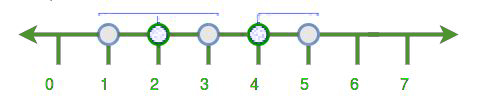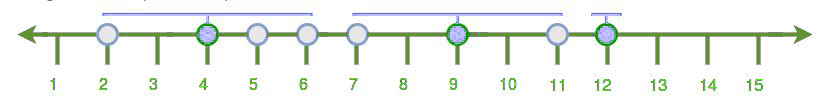Related Articles

# Minimum number of towers required such that every house is in the range of at least one tower

• Difficulty Level : Medium
• Last Updated : 19 Oct, 2021

Given a map of the city and the network range, the task is to determine the minimum number of the tower so that every house is within range of at least one tower. Each tower must be installed on top of an existing house.

Examples:

```Input: range : 1
house : 1 2 3 4 5
Output: 2

Input: range : 2
house : 7 2 4 6 5 9 12 11
Output: 3```All cities can be covered by inserting 2 towers i.e. at house 2 and 4.All cities can be covered by inserting 3 towers i.e. at house 4, 9, and 12.
Algorithm:-

1. First, sort all the elements.
2. Count only once and then traverse till its middle house.
3. After this again traverse till tower range.
4. Again repeat 1, 2, 3 steps till all the houses are covered.

Below is the implementation of above approach:

## C++

 `// C++ implementation of above approach``#include ``using` `namespace` `std;` `// Function to count the number of tower``int` `number_of_tower(``int` `house[], ``int` `range, ``int` `n)``{` `    ``// first we sort the house numbers``    ``sort(house, house + n);` `    ``// for count number of twoers``    ``int` `numOfTower = 0;` `    ``// for iterate all houses``    ``int` `i = 0;``    ``while` `(i < n) {` `        ``// count number of towers``        ``numOfTower++;` `        ``// find find the middle location``        ``int` `loc = house[i] + range;` `        ``// traverse till middle location``        ``while` `(i < n && house[i] <= loc)``            ``i++;` `        ``// this is point to middle``        ``// house where we insert the tower``        ``--i;` `        ``// now find the last location``        ``loc = house[i] + range;` `        ``// traverse till last house of the range``        ``while` `(i < n && house[i] <= loc)``            ``i++;``    ``}` `    ``// return the number of tower``    ``return` `numOfTower;``}` `// Driver code``int` `main()``{``    ``// given elements``    ``int` `house[] = { 7, 2, 4, 6, 5, 9, 12, 11 };``    ``int` `range = 2;``    ``int` `n = ``sizeof``(house) / ``sizeof``(house);` `    ``// print number of towers``    ``cout << number_of_tower(house, range, n);``}`

## Java

 `// Java implementation of above approach` `import` `java.util.Arrays;` `public` `class` `Improve {` `    ``// Function to count the number of tower``    ``static` `int` `number_of_tower(``int` `house[], ``int` `range, ``int` `n)``    ``{` `        ``// first we sort the house numbers``        ``Arrays.sort(house);` `        ``// for count number of towers``        ``int` `numOfTower = ``0``;` `        ``// for iterate all houses``        ``int` `i = ``0``;``        ``while` `(i < n) {` `            ``// count number of towers``            ``numOfTower++;` `            ``// find find the middle location``            ``int` `loc = house[i] + range;` `            ``// traverse till middle location``            ``while` `(i < n && house[i] <= loc)``                ``i++;` `            ``// this is point to middle``            ``// house where we insert the tower``            ``--i;` `            ``// now find the last location``            ``loc = house[i] + range;` `            ``// traverse till last house of the range``            ``while` `(i < n && house[i] <= loc)``                ``i++;``        ``}` `        ``// return the number of tower``        ``return` `numOfTower;``    ``}`  `    ` `    ``public` `static` `void` `main(String args[])``    ``{``        ``// given elements``        ``int` `house[] = { ``7``, ``2``, ``4``, ``6``, ``5``, ``9``, ``12``, ``11` `};``        ``int` `range = ``2``;``        ``int` `n = house.length;` `        ``// print number of towers``        ``System.out.println(number_of_tower(house, range, n));` `        `  `    ``}``    ``// This code is contributed by ANKITRAI1``}`

## Python 3

 `# Python 3 implementation of``# above approach` `# Function to count the``# number of tower``def` `number_of_tower(house, r, n):` `    ``# first we sort the house numbers``    ``house.sort()` `    ``# for count number of twoers``    ``numOfTower ``=` `0` `    ``# for iterate all houses``    ``i ``=` `0``    ``while` `(i < n) :` `        ``# count number of towers``        ``numOfTower ``+``=` `1` `        ``# find find the middle location``        ``loc ``=` `house[i] ``+` `r` `        ``# traverse till middle location``        ``while` `(i < n ``and` `house[i] <``=` `loc):``            ``i ``+``=` `1` `        ``# this is point to middle``        ``# house where we insert the tower``        ``i ``-``=` `1` `        ``# now find the last location``        ``loc ``=` `house[i] ``+` `r` `        ``# traverse till last house``        ``# of the range``        ``while` `(i < n ``and` `house[i] <``=` `loc):``            ``i ``+``=` `1` `    ``# return the number of tower``    ``return` `numOfTower` `# Driver code``if` `__name__ ``=``=` `"__main__"``:``    ` `    ``# given elements``    ``house ``=` `[ ``7``, ``2``, ``4``, ``6``, ``5``, ``9``, ``12``, ``11` `]``    ``r ``=` `2``    ``n ``=` `len``(house)` `    ``# print number of towers``    ``print``(number_of_tower(house, r, n))` `# This code is contributed``# by ChitraNayal`

## C#

 `// C# implementation of above approach` `using` `System;` `public` `class` `Improve {` `    ``// Function to count the number of tower``    ``static` `int` `number_of_tower(``int` `[]house, ``int` `range, ``int` `n)``    ``{` `        ``// first we sort the house numbers``        ``Array.Sort(house);` `        ``// for count number of towers``        ``int` `numOfTower = 0;` `        ``// for iterate all houses``        ``int` `i = 0;``        ``while` `(i < n) {` `            ``// count number of towers``            ``numOfTower++;` `            ``// find find the middle location``            ``int` `loc = house[i] + range;` `            ``// traverse till middle location``            ``while` `(i < n && house[i] <= loc)``                ``i++;` `            ``// this is point to middle``            ``// house where we insert the tower``            ``--i;` `            ``// now find the last location``            ``loc = house[i] + range;` `            ``// traverse till last house of the range``            ``while` `(i < n && house[i] <= loc)``                ``i++;``        ``}` `        ``// return the number of tower``        ``return` `numOfTower;``    ``}`  `    ` `    ``public` `static` `void` `Main()``    ``{``        ``// given elements``        ``int` `[]house = { 7, 2, 4, 6, 5, 9, 12, 11 };``        ``int` `range = 2;``        ``int` `n = house.Length;` `        ``// print number of towers``        ``Console.WriteLine(number_of_tower(house, range, n));` `        ` ` ``// This code is contributed by inder_verma..``    ``}``    ` `}`

## PHP

 ``

## Javascript

 ``
Output:
`3`

Time Complexity: O(nlogn)
Space Complexity: O(1)

Attention reader! Don’t stop learning now. Get hold of all the important DSA concepts with the DSA Self Paced Course at a student-friendly price and become industry ready.  To complete your preparation from learning a language to DS Algo and many more,  please refer Complete Interview Preparation Course.

In case you wish to attend live classes with experts, please refer DSA Live Classes for Working Professionals and Competitive Programming Live for Students.

My Personal Notes arrow_drop_up